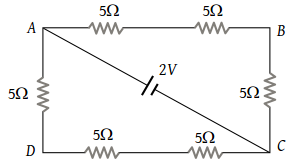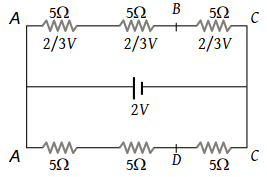# NEET Questions Solved

PMT - 1991

The potential difference between points A and B of adjoining figure is(1) $\frac{2}{3}V$

(2) $\frac{8}{9}V$

(3) $\frac{4}{3}V$

(4) 2 V

(3) The given circuit can be redrawn as followsFor identical resistances, potential difference distributes equally among all. Hence potential difference across each resistance is $\frac{2}{3}V$ and potential difference between A and B is $\frac{4}{3}V.$

Difficulty Level:

• 20%
• 23%
• 41%
• 18%
Crack NEET with Online Course - Free Trial (Offer Valid Till August 23, 2019)